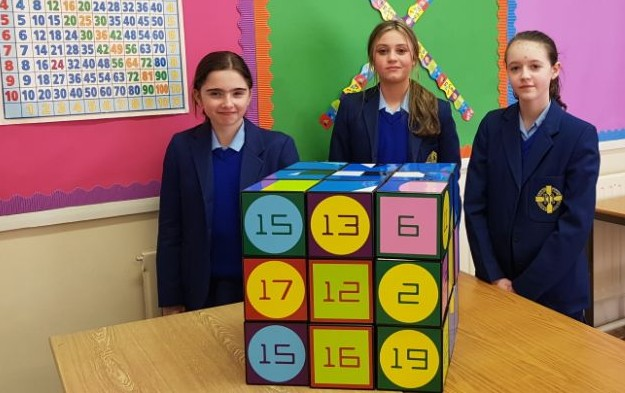## Welcome to the Maths DepartmentDepartment Staff

Mrs L. Dolan (Head of Department)

Mrs L. Kearney

Mr D. Wylie

Ms E. Owens

Ms R. Flynn

Introduction

At Key Stage 3, Mathematics and Numeracy has two subject strands: Mathematics and Financial Capability. This gives pupils a wider understanding of how the world around them works and prepares them for making decisions, solving problems and processing everyday information.

Mathematics helps pupils to develop important skills such as calculating, logical thinking and investigating. It enables pupils to:

• understand quantity and measures;
• appreciate patterns and relationships;
• explore size and shape; and
• analyse data and chance.

Through Financial Capability, pupils are given opportunities to develop knowledge and understanding of a range of finance issues. Pupils should also develop skills that enable them to make competent and responsible financial decisions.

By engaging with issues that young people can relate to, now and in the future, teachers can help pupils to see the relevance of Mathematics and Financial Capability to real life and to work.

## GCSE Mathematics

GCSE Mathematics is a modular exam which is examined through the CCEA Examinations Board.

This course requires more problem-solving and applying mathematics than previous specifications

and introduces functional elements in mathematics.

The course gives students opportunities:

• to develop their knowledge, skills and understanding of mathematical methods and concepts
• to acquire and use problem-solving strategies
• to select and apply mathematical techniques and methods in mathematical, everyday and real-world situations
• to reason mathematically, make deductions and inferences, and draw conclusions
• to interpret and communicate mathematical information in a variety of forms
• for progression, including progression to further study of mathematics at AS/A2 level.

The units all provide opportunities for students to develop and apply their mathematical skills to real-life contexts.  Pupils choose one unit in Year 11 and one unit in Year 12.  The grades that students can achieve depend on the units that they sit for assessment.

In Year 11 pupils choose one unit from:

• Unit M1 or M2 Foundation Tier
• Unit M3 or M4 Higher Tier
Year 11   Foundation Unit M1 or M2   45%

Calculator Written Paper  1 hour 45 minutes in the form of a single question and answer booklet that includes a formula sheet.

Calculator Written Paper  1 hour 45 minutes in the form of a single question and answer booklet that includes a formula sheet.

Year 11   Higher Unit M3 or M4   45%

Calculator Written Paper  2 hours in the form of a single question and answer booklet that includes a formula sheet.

M4 Grades Available A* - C

Calculator Written Paper  2 hours in the form of a single question and answer booklet that includes a formula sheet.

In Year 12 pupils sit one unit.

Year 12  Foundation Unit M5 or M6 55%

Written papers two  1 hour in the form of a question and answer booklet that includes a formula sheet.

1 calculator and 1 non- calculator papers

M6 grades Available C* - G

Written papers two 1 hour 15 minutes in the form of a question and answer booklet that includes a formula sheet.

1 calculator and 1 non- calculator papers

Year 12   Higher Unit M7 or M8 55%

Written papers two 1 hour 15 minutes in the form of a question and answer booklet that includes a formula sheet.

1 calculator and 1 non- calculator papers